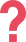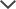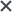My 3rd grade daughter was recommended to take the CogAT. We loved the explanations as they helped her to understand the material. The packs are a helpful tool and less expensive than a private tutor.
Aminata
My son was preparing for CogAT Level 9. With practice, my son got used to the question styles and the practice built his confidence with the exam. Once kids know they can do well, there is no limit – they will always do their best. I have recommended TestPrep-Online to all my friends.
A.A.
We got the CogAT tests for my daughter. I loved how quickly I was able to access the tests after purchasing the product and how easy it was to use! It familiarized my daughter with the format of the test, allowing her to feel calm for the test.
Margaret
We used the CogAT practice pack. The questions in this study pack were very similar in style to what appeared on the actual test. I would definitely recommend this product to other parents.
Ryan
The CogAT test pack provided my son with challenging questions, which prepared him to perform well on the test.
Subra
Worth it. My son was taking the CogAT Level 5/6 for school and with help from the practice tests, he scored well and was able to get into the county's gifted program. Thank you so much.
Oliver
We used the CogAT practice tests and I would definitely recommend it to other parents. I liked that my child had both the timed and step-by-step options when taking a practice test.
Lisa
We got the CogAT practice tests for our daughter. She nailed the test and I would totally recommend TestPrep-Online to other parents.
Jonathan

## CogAT Summary

The CogAT test is composed of three batteries known as the Verbal Battery, Nonverbal Battery, and Quantitative Battery. Each battery is then divided into three sub-tests that measure different skills and require different reasoning.

Below you will find three sample questions belonging to each of the three batteries.The first question below belongs to the verbal battery of the CogAT. You are presented with three words belonging to a certain group. One of the answer choices displays a word that belongs to the same group as the other three words. The idea is for you to extract the information about the group, and locate the answer that belongs in that same category.

The second question is a classic example of the Quantitative Battery. You are presented with a system of equations but expressed in a simple way. Two numbers are missing from the system, one of them can be calculated directly and the other number depends on the first result. This question measures your arithmetic, analytical skills, and overall ability to understand that the symbols displayed represent numbers and how to calculate them. You can also approach this question by using the answer choices. These and other strategies are available in our practice packs. After you have seen a few of these questions you will be able to solve all of them quickly.

The third question is commonly referred to as a "paper folding" exercise, and it is part of the Non-Verbal Battery. In these questions, a blue square is usually presented as a piece of paper, it is then folded, and finally pierced in some places. The question can be interpreted as: if you unfold the paper after you have pierced it, which of the answer choices represents the resulting shape? In order to solve this, you need spatial visualization and a bit of imagination. However, you can also learn a good trick from our practice packs. If you fold a paper in four, and pierce it twice, the number of holes it should have is 2 x 4 = 8 (two holes for each time it was folded). Therefore, many answer choices can be ruled out simply by counting the holes without even requiring spatial visualization.

All of the questions in the practice pack include detailed explanations with logical, intuitive strategies and other interesting tricks.

## Sample Test PreviewThink about how the words in the top row go together. Which word in the bottom row fits best with the words in the top row?Which number goes in the square with the question mark?Look at the top row. See how the paper is folded and changed. Fill in the circle under the picture that shows how the paper looks when it is opened.

 Choice 4 – The correct answer is: lion These are all animals belonging to the biological family of cats.

 Choice 3 – The correct answer is 21. This question has two equations. In both equations, both sides of the equal sign must total the same amount. Since we are trying to solve for the ‘?’, let’s use the information the second equation gives us to help us solve the first equation. The second equation tells us that ◊ = 12 ÷ 4, which means that ◊ = 3. When we replace the diamond in the first equation with 3, we get: ? – 6 = 5 × 3. To find out what the ‘?’ stands for, let’s first solve the right side of the equation: 5 × 3 = 15, so: ? – 6 = 15. This means that ? = 21, because 21 – 6 = 15. Therefore, 21 is the correct answer.

 Choice 5 – The square paper was first folded in half diagonally to create a triangle, and then folded again from each corner towards the middle of the triangle. Then, four holes were cut out of the folded paper. All four holes go through four layers of paper. Therefore, the answer should have sixteen holes (4 holes x 4 layers). The holes will flip over the fold each time the paper is unfolded – first upwards and to the left, and then diagonally. Answer choices 1, 2, and 3 include less than 16 holes, so they can be eliminated. Answer choice 4 does not mirror correctly the holes in the folded paper above. We are left with the 5th answer choice, which is the correct answer.

TestPrep-Online offers:

• Level specific, full-length practice tests
• Hundreds of practice questions
• Study guides for children
• Parent manuals

## Looking For Related CogAT Free Sample Tests?

-Free CogAT kindergarten sample test

-Free CogAT 1st grade sample test

-Free CogAT 2nd sample test

-Free CogAT 3rd grade sample test

-Free CogAT 4th grade sample test

-Free CogAT 5th grade sample test

The CogAT and other trademarks are the property of their respective trademark holders. None of the trademark holders are affiliated with TestPrep-Online or this website.

Reviews

Need HelpNeed Help